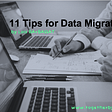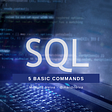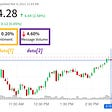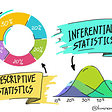# A/B Testing with Statistical Inference

The A/B testing is commonly used nowadays for advertisement. Two customers can visit a retail webpage, and each sees different content.
The goal here is to select to measure which advertisement is effective.

What if the proportions are different? What if advertisement A got 400 clicks and advertisement B got 360 clicks. Which one do you choose, and how confident are you?

The question becomes:
Is there a significant difference between the population proportions of the population a and population b?

`Null hypothesis (H0):        pa — pb = 0Alternative hypothesis (Ha): pa — pb ≠ 0Alpha*:                      0.05* The threshold we use to decide if the p-value is sufficiently low to reject the null hypothesis.`

Since the views are independent, and we can view each as a Bernoulli trial, we can generate that data as follows:

`n = 1000pa = 400 / npb = 360 / npopulation1 = binom.rvs(1, pa, size=n)population2 = binom.rvs(1, pb, size=n)ttest_ind(population1, population2)#output: (statistic=0.09168604722034365, pvalue=0.9269566745658202)`

The t-test score is 0.092, and the p-value is 0.92.
Since 0.92 > 0.05, we can’t reject the null hypothesis, which means there is no statistical significance to favor one advertisement over the other.

`population2 = binom.rvs(1, pb, size=n)ttest_ind(population1, population2)#output: (statistic=2.135431894379663, pvalue=0.03284703858914734)`

The t-test score is 2.135, and the p-value is 0.033.
Since 0.033 < 0.05, we can reject the null hypothesis, which means there is a statistical significance to favor advertisement a.

As you can see, statistics has powerful tools to make better decisions with confidence. Thus when in doubt, t-test it.

https://github.com/booletic/medium/blob/c2b61abf1509c808935ec15501c15e2a441695df/ab_testing.ipynb

--

--

--

## More from Mansoor Aldosari

Reject the null hypothesis

Love podcasts or audiobooks? Learn on the go with our new app.

## Lessons learned by a rookie data scientist from working in a real data team.## The Great Hack — Approach Outline## 11 Tips for Data Migrations## 5 queries you must know in SQL## Halfway Through a Data Science Bootcamp## Stock Sentiment Analysis with Python — Stocktwits## Data-Driven Government in Large & Small Cities## Data vs Traffic Warden## Mansoor Aldosari

Reject the null hypothesis

## Inferential Statistics in Data Science## IoT Analytics## Performing Analysis of Meteorological Data## How to perform Exploratory Data Analysis (EDA) and clean your data for model training?